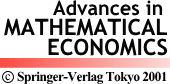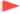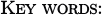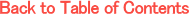Adv. Math. Econ. Volume 3, pp.63-82 (2001)

Recursive method in stochastic optimization under compound criteria

Seiichi Iwamoto
Department of Economic Engineering, Graduate School of Economics, Kyushu University 27, Hakozaki 6-19-1, Higashiku, Fukuoka 812-8581, JapanIn this paper we propose a recursive method in stochastic optimization problems with compound criteria. By introducing four (Markov, general, primitive and expanded Markov) types of policy, we establish an equivalence among three (general, expanded Markov and primitive) policy clatsSes. It is shown that there exists an optimal policy in general class. Further we apply this result to range, ratio and variance problems. We derive both forward recursive formula for past-value sets and backward recursive formula for value functions. The compound criteria is large for economic decision processes.dynamic programming, invariant imbedding, compound criteria, backward recursive formula, forward recursive formula, past-value sets, expanded Markov policy, general policy, primitive policy, range, ratio, variance